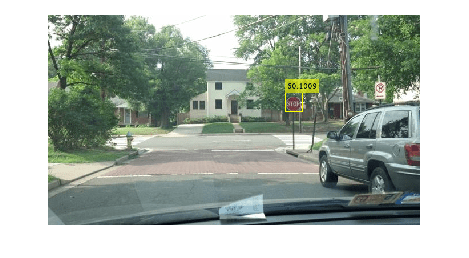# Generate Code for Detecting Objects in Images by Using ACF Object Detector

This example shows how to generate code from a MATLAB function that detects objects in images by using an `acfObjectDetector` object. When you intend to generate code from your MATLAB function that uses an `acfObjectDetector` object, you must create the object outside of the MATLAB function. The example explains how to modify the MATLAB code in Train a Stop Sign Detector Using an ACF Object Detector to support code generation.

### Design the MATLAB Code File for Code Generation

To generate C Code, MATLAB Coder requires MATLAB code to be in the form of a function. The arguments of the function cannot be MATLAB objects. This requirement presents a problem for generating code from the MATLAB function that uses `acfObjectDetector` objects created outside of the MATLAB function. To solve this problem, use the `toStruct` function to convert the `acfObjectDetector` object into a structure and pass the structure to the MATLAB function.

To support code generation, this example restructures the code of an existing example ( See Train a Stop Sign Detector Using an ACF Object Detector) in a function called `detectObjectsUsingACF`, which is present in the current working folder as a supporting file. The `detectObjectsUsingACF` function takes an image as an input and loads the pretrained ACF stop sign detector.

`type('detectObjectsUsingACF.m')`
```function [bboxes,scores] = detectObjectsUsingACF(InputImage) % Load a trained detector from a MAT file S = coder.load('detectorStruct.mat'); % Define a persistent variable persistent detector if isempty(detector) % Re-create the ACF Object Detector detector = acfObjectDetector(S.detectorStruct.Classifier,S.detectorStruct.TrainingOptions); end % Use the detect function to detect objects in the input image [bboxes,scores] = detect(detector,InputImage); end ```

### Create ACF Stop Sign Detector Outside of the MATLAB Function

`load('stopSignsAndCars.mat')`

Select the ground truth for stop signs. The ground truth data is the set of known locations of stop signs in the images.

`stopSigns = stopSignsAndCars(:,1:2);`

Add the full path to the image files.

```stopSigns.imageFilename = fullfile(toolboxdir('vision'),... 'visiondata',stopSigns.imageFilename);```

Use the `trainACFObjectDetector` function to train the ACF detector. Turn off the training progress output by setting `'Verbose' to false`.

`detector = trainACFObjectDetector(stopSigns,'NegativeSamplesFactor',2,'Verbose',false); `

### Generate C-MEX Function

Because you intend to generate code for the MATLAB function `detectObjectsUsingACF`, convert the created `detector` into a structure.

`detectorStruct = toStruct(detector);`

Save the trained object structure as a MAT file.

`save('detectorStruct.mat','detectorStruct'); `

Generate C-MEX code that you can run in the MATLAB environment. Use the `codegen` (MATLAB Coder) command.

`codegen detectObjectsUsingACF -report -args { coder.typeof(uint8(0), [inf inf 3])}`
```Code generation successful: To view the report, open('codegen/mex/detectObjectsUsingACF/html/report.mldatx') ```

### Detect Objects Using Generated C-MEX Function

To detect objects in an image, load a test image.

`img = imread('stopSignTest.jpg');`

Call the generated C-MEX function by passing the loaded image `img` as an input.

`[bboxes, scores] = detectObjectsUsingACF_mex(img);`

Display the detection results and insert the bounding boxes for objects into the image.

```img = insertObjectAnnotation(img,'rectangle',bboxes,scores); figure imshow(img)```### Clean Up

Release the system memory used to store the generated C-MEX file.

`clear ObjectDetectionFromImages_mex;`